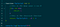# Call Me By My Name…. Recursion

If you are new to programming, or even if you aren't, you have probably heard of a type of algorithm called Recursion and freaked out. This is normal and with time you will soon realize that the name may sound daunting, but it is really quite simple.

The simplest way to think of recursion is by using an analogy like a Russian Doll set. In order to get the the smallest doll, you have to continue to go into itself and see if there is anything else within it. Finally, when you get to the inner most doll, you can take it out and place all the other ones back in, one by one.

# How does that analogy fit in with programming?

Well, let’s look at an example of how recursion would be used to solve a problem.

Recursion has 2 parts to the algorithm, a recursive call and a base case. The recursive call is the function that is going to be repeatedly called within itself, while the base case is the stopping point at which you want your function to stop calling itself. IMPORTANT: Make sure you have a base case. Without it, your program will crash due to an infinite loop.

So let’s use a math function like factorials. If you are a little rusty in math, that is fine, we will briefly go over factorials to refresh your memory. Factorials are the product of all the positive integers from 1 to n. Factorials are denoted by the given integer along with an exclamation point (i.e. 5!). So 5! would be 5 * 4 * 3 * 2 * 1, which equals 120.

Ok, now that we quickly refreshed ourselves, let’s dive into the example.Recursive example

Let’s break this function down to understand what is going on. We have a function called factorial, which accepts a number, n. While n is greater than 0, the function will call itself, subtracting 1 from the current value of n each time till it equals 0.

The above screenshot shows how the function works each time it dives deeper into itself. Let’s start at line 16. We make a call to the function called factorial with the number 5 as the argument. Since 5 is not equal to 0 (line 4), it skips that command and calls itself (line 8), except now subtracting 1 from 5. You can also see the function being returned is also being multiplied by n.

When we finally hit 0, we return 1 (line 5). Now is where all the math begins. The function has went 5 levels within itself before hitting 0 and now works its way back up.

Starting at line 18, we see that 1 is multiplied by the function factorial(0) which is 1, then it moves up to line 16. Factorial(1) now is multiplied by 2, which return 2. It does this all the way back up till we get to our top level which was when n === 5. The returned result would be 120.

Hopefully this helped clear up any confusion you had about Recursion. The best way to learn is by doing. So try solving some problems using recursion and over time you will get the hang of it!

Full Stack Software Engineer. Bachelor’s in Technology —Specializing in Software Development from NYCCT — CUNY. Flatiron School Graduate

## More from David Kirsch

Full Stack Software Engineer. Bachelor’s in Technology —Specializing in Software Development from NYCCT — CUNY. Flatiron School Graduate